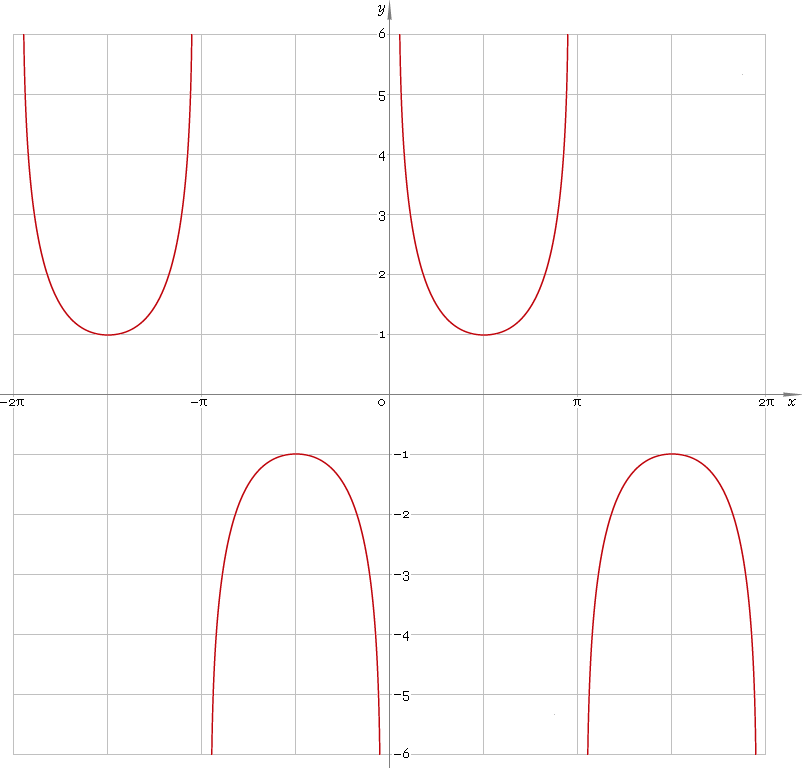The Art of Mathematics

# csc or cosec — trigonometric cosecant function

## 1. Definition

Cosecant of the angle is ratio of the hypotenuse to opposite leg.

## 2. Graph

Cosecant is 2π periodic function defined everywhere on real axis, except its singular points πn, n = 0, ±1, ±2, ... — so function domain is (πn, π(n + 1)), n∈N. Its stalactite-stalagmite graph is depicted below — fig. 1.Fig. 1. Graph of the cosecant function y = cscx.

Function codomain is entire real axis with a gap in the middle: (−∞, −1]∪[1, +∞).

## 3. Identities

Base:

csc−2φ + sec−2φ = 1

and its consequences:

cscφ = ±1 /√(1 − cos2φ)
cscφ = ±√(1 + tan2φ) / tanφ
cscφ = ±√(1 + cot2φ)
cscφ = ±secφ /√(sec2φ − 1)

By definition:

cscφ ≡ 1 /sinφ

Properties — symmetry, periodicity, etc.:

csc−φ = −cscφ
cscφ = csc(φ + 2πn), where n = 0, ±1, ±2, ...
cscφ = csc(π − φ)
cscφ = −csc(π + φ)
cscφ = sec(π/2 − φ)

Sum of angles:

csc(φ + ψ + χ) = secφ secψ secχ / (tanφ + tanψ + tanχ − tanφ tanψ tanχ)

Some angles:

Angle φValue cscφ
π/12√6 + √2
π/10√5 + 1
π/8√(4 + 2√2)
π/62
π/5√(50 + 10√5) /5
π/4√2
3π/10√5 − 1
π/32√3 /3
3π/8√(2 − √2)
2π/5√(50 − 10√5) /5
5π/12√6 − √2
π/21
Table 1. Cosecant for some angles.

## 4. Derivative and indefinite integral

Cosecant derivative:

csc′x = −cscx cotx ≡ −cosx /sin2x

Indefinite integral of the cosecant:

∫ cscx dx = ln|cscx − cotx| + C = ln|tan(x/2)| + C

where C is an arbitrary constant.

## 5. How to use

To calculate cosecant of the number:

``csc(−1);``

To get cosecant of the complex number:

``csc(−1−i);``

To get cosecant of the current result:

``csc(rslt);``

To get cosecant of the angle φ in calculator memory:

``csc(mem[φ]);``

## 6. Support

Trigonometric cosecant of the real argument is supported in free version of the Librow calculator.

Trigonometric cosecant of the complex argument is supported in professional version of the Librow calculator.